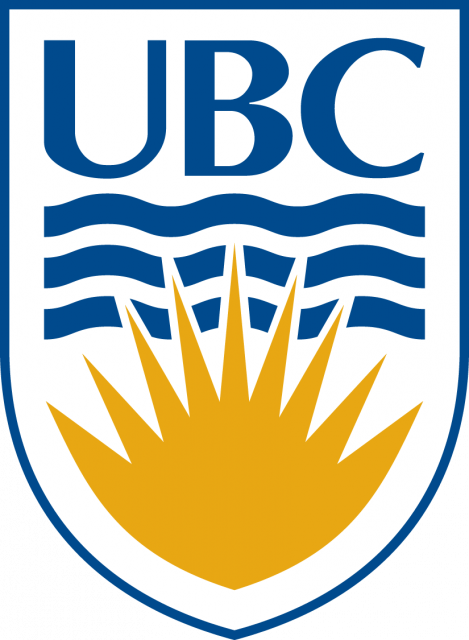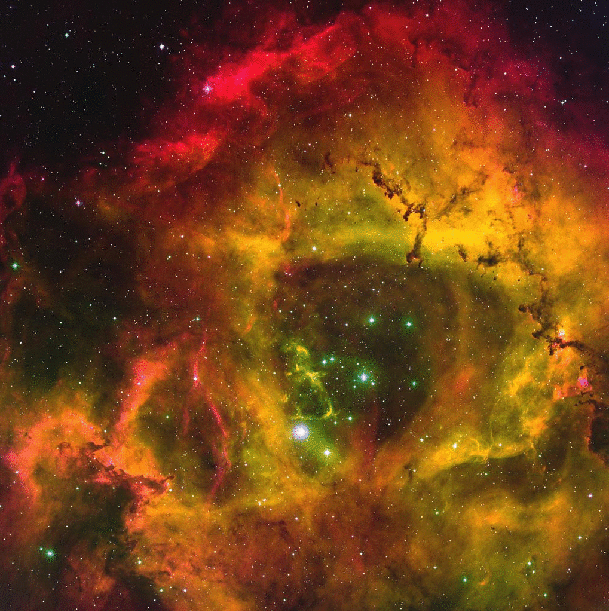Silvestre Aguilar-Martinez
University of British Columbia

# Research:

My current research projects focus on the physics of compact objects which are of great significance in astrophysics, examples of these are, black holes, neutron stars, white dwarfs, and degenerate stars. Much can be learned in regards to the interaction of matter+energy and gravity from studying the formation dynamics, and evolution of these objects. They however, generally constitute extreme states of matter whose condition are not easily replicable in a laboratory setting. Due to this fact computation approaches have emerged which in principle allow for their replication in the form of computer simulations thus providing a vehicle for their investigation.Our particular goal is to investigate these exotic states of matter using computational techniques. This means solving Einstein's equations along with the equations governing the dynamics of the matter fields by implementation of appropriate numerical methods. Often, useful information can be extracted by considering Newtonian gravity as a model for gravitation. In both of these cases the dynamical system is comprised by a set of time-dependent partial differential equations whose solution represent the evolution of both the gravitational and matter fields.

For our description of compact objects to be complete a certain matter-model needs to be provided. Arguably, the most realistic (yet highly idealized) model for the matter fields is the $$perfect$$ $$fluid$$. In this case the fields $$i.e.$$ the dynamical variables are the pressure $$P$$, energy density $$\rho$$, and fluid velocity $$\vec{v}$$. In addition, this model requires a thermodynamics relation, called the equation of state to provide sufficient condtions for the system to be in principle soluble. This simplified fluid description serves as a basic model describing the dynamics of neutron stars.

Our interest in the perfect fluid model stems for theoretical considerations concerning its behavior under conditions of gravitational collapse. Thus our interest is not driven by current observational data but rather by the theoretical/mathematical structures of the theory and equations. More specifically, we are interested in the particular form the physical fields take under certain initial conditions which have come to be called $$critical$$ $$collapse$$. When a self-gravitating fluid is fine tuned to the threshold of gravitational collapse (critical collapse conditions), the fluid flow evolves to a special self-similar state with special properties. Upon close examination of the critical solution one discovers a structure that is reminiscent of critical phase transitions in statistical mechanics. Given this parallel nature with statistical physics this structure has been called $$critical$$ $$phenomena$$ and was first discovered by Choptuik (1993) in critical collapse simulations of a real scalar field in General Relativity. Critical phenomena has wide spread implications in the areas of cosmic censorship and quantum gravity and arises in various matter models, here we are only concerned with the perfect fluid.

# Critical Collapse of Newtonian Fluids: the role of angular momentum

last update: Tue Jul 21, 2015